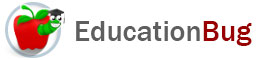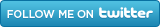PUBLIC SCHOOLS PRIVATE SCHOOLS SCHOOL DISTRICTS COLLEGES PUBLIC LIBRARIES JOBS BLOG RESOURCES
Career Education
Education Choices
Education Costs
Education History and General Information
Education Issues
Education Resources
Family Involvement in Education
Students w Special Needs
Subject Information and Homework Help
Teaching and Learning
Testing
By Types of Schools
By Specific Audiences
GeneralDecimals

Decimals are numbers written using the decimal system. The decimal system is based on powers of 10 and assigned places, so that the position of a digit with respect to the decimal point is indicative of its value. For more about decimals, keep reading.

Uniqueness of the Decimal System

Have you ever used tally marks to count something? The tally system usually includes four straight, vertical marks with the fifth mark crossing all four diagonally. If itâ€™s necessary to use another group, one again makes up to four straight, vertical marks, and, if necessary, a fifth mark crossing that group of four diagonally.

In the tally system, no mark is different than any other mark. Each represents the same thing - one of whatever is being represented - no matter whether its straight and vertical or diagonal, no matter where on the page it is drawn.

Not so in the decimal system. The reason that the decimal system can do so much with only the ten digits 0, 1, 2, 3, 4, 5, 6, 7, 8, 9 is that assigning meaning to â€œplacesâ€ to the left and the right of an indicator that looks like a period but is called a decimal point allows expression of many, many numbers in an orderly and easily manipulated way.

How the Decimal System Works

This is a description of how the decimal system works. Places increase in value to the left of the decimal point by multiples of ten and decrease in value to the right of the decimal point by multiples of ten. The places have these names moving left from the decimal point:

ones, tens, hundred, thousands, ten thousands, hundred thousands, millions, ten millions, hundred millions, billions, etc.

The places have these names moving right from the decimal point:

tenths, hundredths, thousandths, ten thousandths, hundred thousandths, millionths, ten millionths, hundred millionths, billionths, etc.

Any empty places between the farthest left digit to the left of the decimal point and the decimal point itself and any empty places between the farthest right place when working to the right of the decimal point and the decimal point itself must be filled by a zero, which functions as a place holder. So if you want to write 3 million, you have to write:

3,000,000

and if you want to write 3 millionths, you have to write:

0.0000003

If you wanted to write 24 hundredths, youâ€™d be sure that the farthest right digit was in the hundredths place, like this:

0.24

Numbers written solely to the right of the decimal point, like 3 millionths or 24 hundredths are casually called decimals. Decimals cannot be written without a decimal point, and in some styles, they must also include a zero in the oneâ€™s place as a place holder, as shown above.

A single digit, written by itself, is assumed to be in the ones place. In fact, any group of digits, written without the decimal point are assumed to be interpretable based on the farthest right digit being in the ones place. Sometimes these digits are separated into groups of three by interposed commas, which may make long numbers easier to read.

Different Representations of Decimals

Decimals can also be represented as fractions by putting the digit value in the numerator and the numeral form of the place name in the denominator. So, for example, with 3 millionths, or 0.0000003, youâ€™d put the 3 in the numerator and 1 million in the denominator, like this:

3/1,000,000

If you started with .24, youâ€™d have 24/100, and so on.

Decimals can also be represented as percentages by moving the decimal point two places to the right, eliminating any zeroes to the left of the decimal point, and using the percent sign or the word percent. So, to keep using our example of 3 millionths, youâ€™d have this:

.00003%

If you started with .24, youâ€™d have 24%, and so on.

Related Articles
Subtraction Multiplication